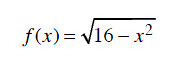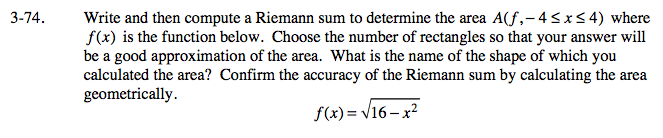### Home > CALC > Chapter 3 > Lesson 3.2.3 > Problem3-74

3-74.

Write and then compute a Riemann sum to determine the area A(f, − 4 ≤ x ≤ 4) where f(x) is the function below. Choose the number of rectangles so that your answer will be a good approximation of the area. What is the name of the shape of which you calculated the area? Confirm the accuracy of the Riemann sum by calculating the area geometrically. Homework Help ✎General form of a left-endpoint Riemann sum:

Hint for choosing the number of rectangles, n: While you want to choose a large value for n, be mindful that you will use that value to compute Δx.

Since ba = 8, it is recommended (though not necessary) that you choose an n that is divisible by 8.

You should have recognized that this function is a semi-circle with center at the origin and radius 4. The exact area can be found with geometry.

Use the eTool below to visualize this problem.
Click the link at right for the full version of the eTool: Calc 3-74 HW eTool